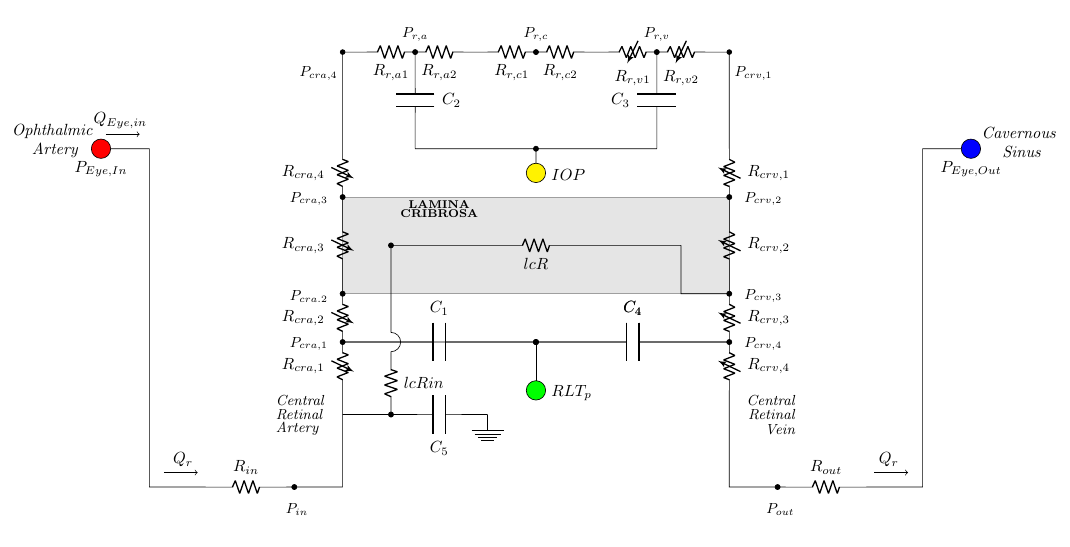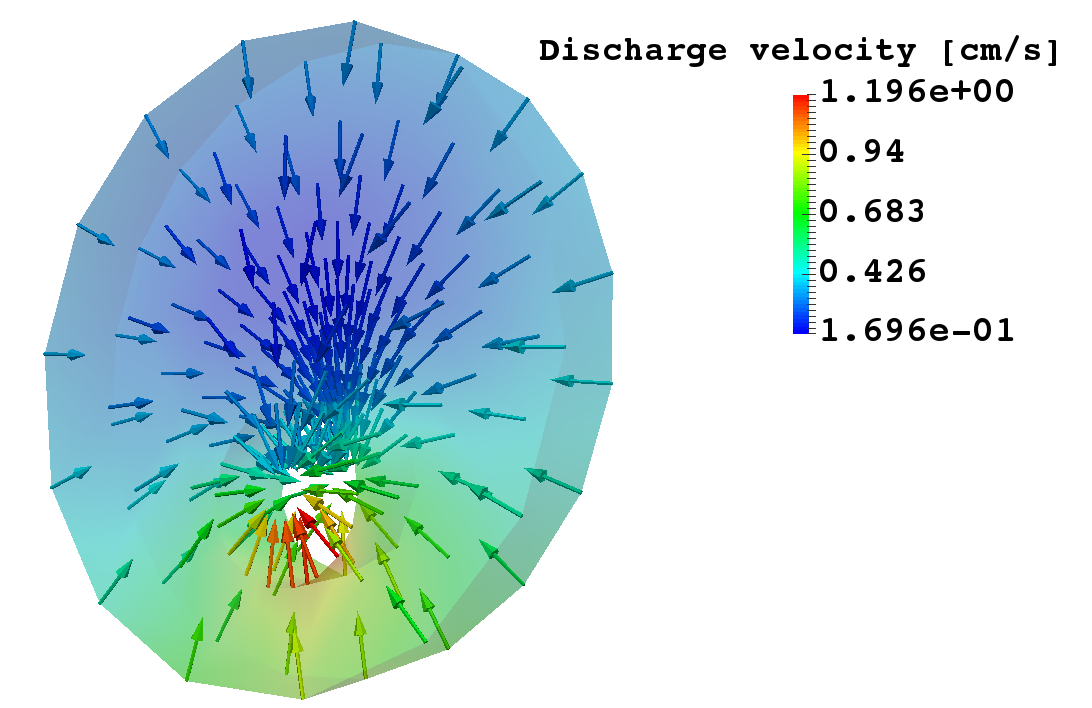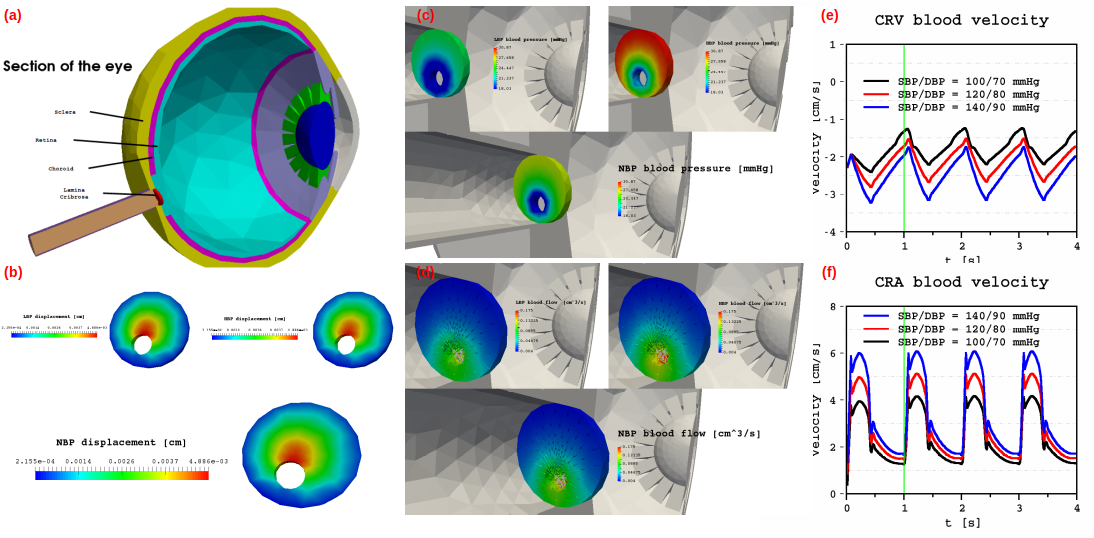Models and Applications

We discuss in this chapter the models and experiments that we conducted within the Eye2Brain project using the Ocular Mathematical Virtual Simulator. We start first by saying a few words about the underlying mathematical framework, then describe the geometrical model and then present the three modelling level already available.

1. Mathematical Methods

Eye2Brain and the Ocular Mathematical Virtual Simulator are based upon advanced mathematical modeling and numerical methods.

3. Level 0Level 0: simulation of the blood circulation in the retinal vessels.

4. Level 1Level 1: simulation of the hemodynamics of the lamina cribrosa and retinal vessels.

5. Level 2Level 2: simulation of the hemodynamics and biomechanics of the lamina cribrosa coupled with the blood circulation in the retinal vessels.